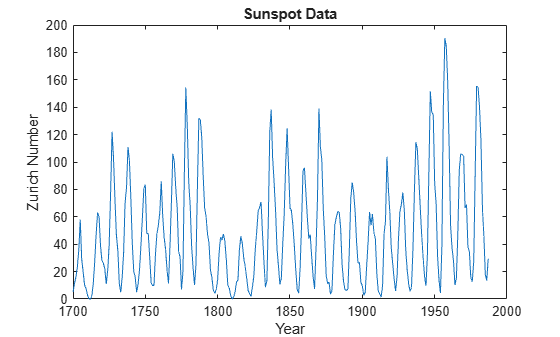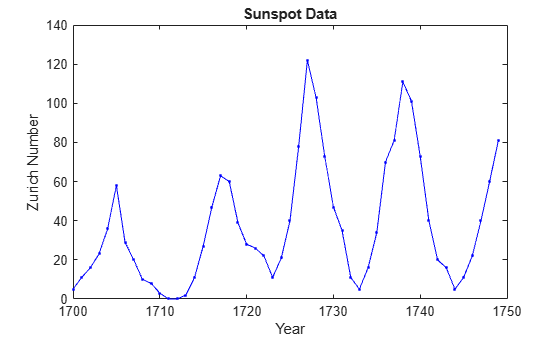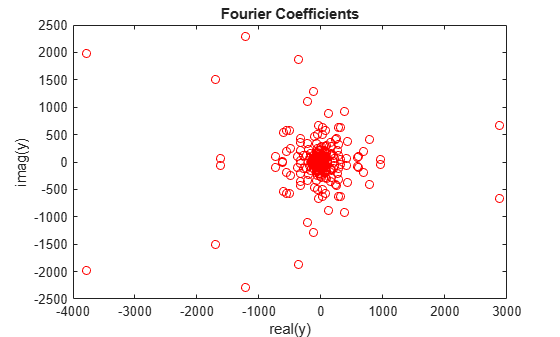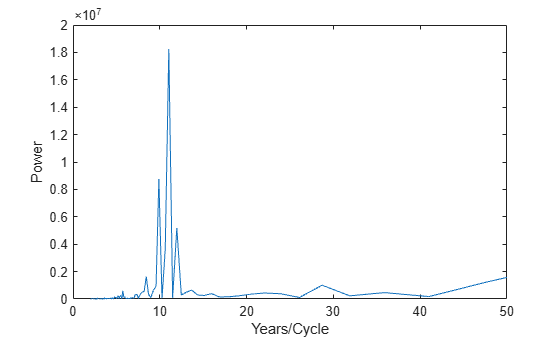Documentation

This is machine translation

Mouseover text to see original. Click the button below to return to the English version of the page.

Analyzing Cyclical Data with FFT

You can use the Fourier transform to analyze variations in data, such as an event in nature over a period time.

For almost 300 years, astronomers have tabulated the number and size of sunspots using the Zurich sunspot relative number. Plot the Zurich number over approximately the years 1700 to 2000.

```load sunspot.dat year = sunspot(:,1); relNums = sunspot(:,2); plot(year,relNums) xlabel('Year') ylabel('Zurich Number') title('Sunspot Data')```To take a closer look at the cyclical nature of sunspot activity, plot the first 50 years of data.

```plot(year(1:50),relNums(1:50),'b.-'); xlabel('Year') ylabel('Zurich Number') title('Sunspot Data')```The Fourier transform is a fundamental tool in signal processing that identifies frequency components in data. Using the `fft` function, take the Fourier transform of the Zurich data. Remove the first element of the output, which stores the sum of the data. Plot the remainder of the output, which contains a mirror image of complex Fourier coefficients about the real axis.

```y = fft(relNums); y(1) = []; plot(y,'ro') xlabel('real(y)') ylabel('imag(y)') title('Fourier Coefficients')```Fourier coefficients on their own are difficult to interpret. A more meaningful measure of the coefficients is their magnitude squared, which is a measure of power. Since half of the coefficients are repeated in magnitude, you only need to compute the power on one half of the coefficients. Plot the power spectrum as a function of frequency, measured in cycles per year.

```n = length(y); power = abs(y(1:floor(n/2))).^2; % power of first half of transform data maxfreq = 1/2; % maximum frequency freq = (1:n/2)/(n/2)*maxfreq; % equally spaced frequency grid plot(freq,power) xlabel('Cycles/Year') ylabel('Power')```Maximum sunspot activity happens less frequently than once per year. For a view of the cyclical activity that is easier to interpret, plot power as a function of period, measured in years per cycle. The plot reveals that sunspot activity peaks about once every 11 years.

```period = 1./freq; plot(period,power); xlim([0 50]); %zoom in on max power xlabel('Years/Cycle') ylabel('Power')```